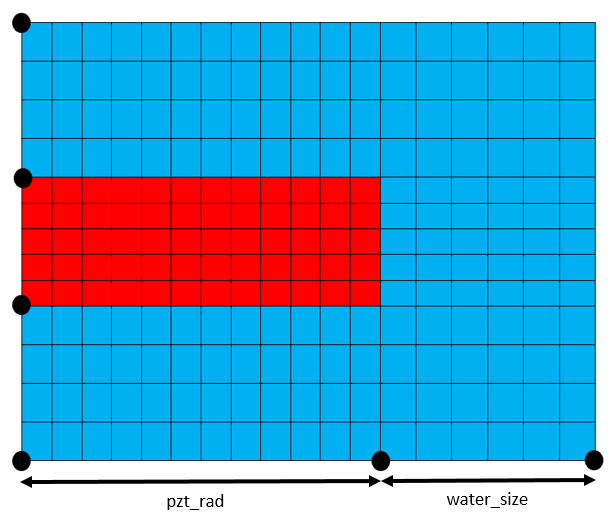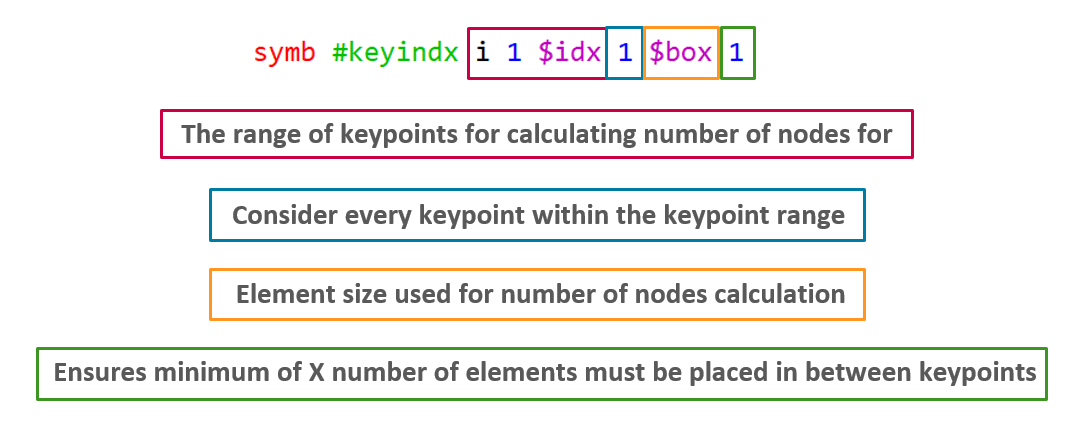# What are #keycord and #keyindx

The symbol #key options allow for a quick method of generating XYZ and IJK model keypoints. The use of these commands also helps to parameterise the model grid generation to allow for quick changes to model geometry to be made.

## #keycord

The #keycord command can be used to generate the XYZ locations of keypoints in the model. For example, the following lines to generate X and Y keypoints:

```symb x1 = 0.
symb x2 = \$x1 + \$pzt_rad
symb x3 = \$x2 + \$water_size

symb y1 = 0.
symb y2 = \$y1 + \$water_size
symb y3 = \$y2 + \$pzt_thk
symb y4 = \$y4 + \$water_size```

can be shorted to:

```symb #keycord x 1 0. \$pzt_rad \$water_size
symb #keycord y 1 0. \$water_size \$pzt_thk \$water_size
```

The below diagram illustrates the keypoints generated in the X direction using #keycord:## #keyindx

The #keyindx command can be used to quickly generate the IJK nodal locations at each keypoint. For example, the following lines:

```symb i1 = 1
symb i2 = \$i1 + nint ( ( \$x2 - \$x1 )  / \$box )
symb i3 = \$i2 + nint ( ( \$x3 - \$x2 )  / \$box )

symb j1 = 1
symb j2 = \$j1 + nint ( ( \$y2 - \$y1 )  / \$box )
symb j3 = \$j2 + nint ( ( \$y3 - \$y2 )  / \$box )
symb j4 = \$j3 + nint ( ( \$y4 - \$y3 )  / \$box )
```

Can be shorted to:

```symb #keyindx i 1 \$idx 1 1
symb #keyindx j 1 \$jdx 1 1```

The #keyindx command inputs is illustrated below: